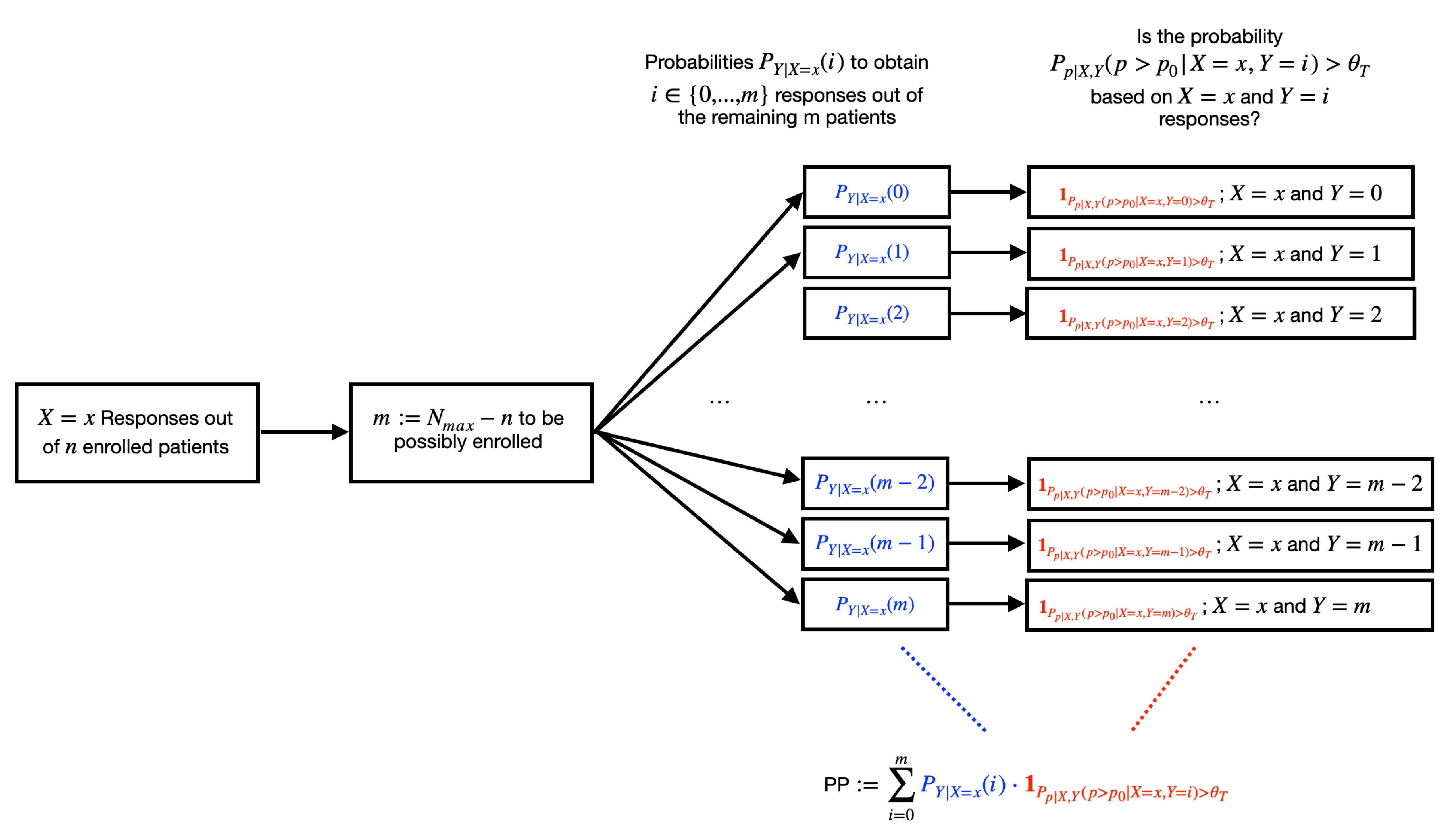# Introduction

In this vignette, the functionality of the brada package is outlined. The brada package provides access to functions which help to plan, analyze and conduct Bayesian response-adaptive (clinical) trial designs. In the current version, the brada package supports only phase IIA trials with a binary endpoint for response or success. Also, the scope of the package is on group-sequential designs, where based on interim analyses the trial can be stopped early for futility or efficacy. In the future, it is planned to include more Bayesian response-adaptive designs in the package.

The outline of the vignette is as follows:

• The first section gives a brief overview about the predictive probability design
• The second section outlines how to get started with the brada package and introduces the core functionality
Note that there are more vignettes which illustrate
• how to apply and calibrate the predictive evidence value design with the brada package. This vignette is hosted at the Open Science Foundation.
• how to monitor a running clinical trial with a binary endpoint by means of the brada package

This vignette builds the starting point, and the vignettes above provide further details.

# The predictive probability design

The general setting considered in is a single-arm phase IIA trial with the goal to evaluate the response rate $$p\geq 0$$ for a new drug or treatment. The null hypothesis $$H_0:p\leq p_0$$ is tested against the alternative $$H_1:p>p_1$$, where $$p_0,p_1\in [0,1]$$, $$p_0 \leq p_1$$ and $$p_0$$ is a predefined threshold for determining the minimum clinically important effect (Kelter, 2021b). For simplicity, assume a Beta prior $$p\sim B(a_0,b_0)$$ is selected for the response rate $$p$$, which offers a broad range of flexibility in terms of modelling the prior beliefs about $$p$$. For the Beta prior, $$a_0/(a_0+b_0)$$ is the mean and $$a_0$$ and $$b_0$$ can be interpreted as the numbers of effective prior responses and non-responses. Thus, $$a_0+b_0$$ gives a measure of how informative the prior is with larger values of $$a_0+b_0$$ indicating a more informative prior distribution.

Let $$N_{\text{max}}$$ be the maximum number of patients which is possibly recruited during the trial, and let $$X$$ be the random variable which measures the number of responses in the current $$n$$ enrolled patients, where $$n\leq N_{\text{max}}$$. A reasonable assumption is that $$X$$ follows a binomial distribution with parameters $$n$$ and $$p$$, $$X\sim \text{Bin}(n,p)$$. The $$B(a_0,b_0)$$ distribution is a conjugate prior for the binomial likelihood, and thus the posterior $$P_{p|X}$$ is also Beta-distributed : $p|X=x\sim B(a_0+x,b_0+n-x)$ The idea of the predictive probability approach consists of analyzing the interim data to project whether the trial will result in a conclusion that the drug or treatment is effective or ineffective. Efficacy is declared when the posterior probability fulfills the constraint $P_{p|X,Y}(p>p_0|X=x,Y=i)>\theta_T$ for some threshold $$\theta_T \in [0,1]$$. That is, when $$n$$ patients have been enrolled in the trial out of which $$X=x$$ show a response, there remain $$m=N_{\text{max}}-n$$ patients which can be enrolled in the trial. If out of these remaining $$m$$ exactly $$i$$ respond to the treatment, and the conditional probability $$P_{p|X,Y}(p>p_0|X=x,Y=i)$$ is larger than a prespecified threshold $$\theta_T$$, say, $$\theta_T=0.95$$, this will be interpreted as the drug being effective. However, as the number $$Y$$ of responses in the remaining $$m=N_{\text{max}}-n$$ patients which can be enrolled in the trial is uncertain, this uncertainty must be modeled, too. Marginalizing out $$p$$ of the binomial likelihood yields the prior predictive distribution which is Beta-Binomial $Y\sim \text{Beta-Binom}(m,a_0+x,b_0+n-x)$ Additionally, from the conjugacy of the beta prior we have the posterior $P_{p|X,Y}(X=x,Y=i)\sim B(a_0+x+i,b_0+N_{\text{max}}-x-i)$ and the expected predictive probability of trial success – henceforth abbreviated $$\text{PP}$$ – can now be calculated by weighing the posterior probability of trial success $P_{p|X,Y}(p>p_0|X=x,Y=i)>\theta_T$ when observing $$X=x$$ and $$Y=i$$ with the prior predictive probability $$P_{Y|X}(Y=i|X=x)$$ of observing $$Y=i$$ responses in the remaining $$m=N_{\text{max}}-n$$ patients, after $$X=x$$ responses have been observed among the current $$n$$ patients: $\text{PP}=\mathbb{E}\left [ 1_{P_{p|X,Y}(p>p_0|X,Y)>\theta_T}|x\right ]=\int_{\mathcal{Y}}1_{P_{p|X,Y}(p>p_0|X,Y)>\theta_T}dP_{Y|X=x}=\sum_{i=0}^m P_{Y|X=x}(i)\cdot 1_{P_{p|X,Y}(p>p_0|X=x,Y=i)>\theta_T}$ where $1_{P_{p|X,Y}(p>p_0|X=x,Y=i)>\theta_T}:=\begin{cases} 1, \text{ if } P_{p|X,Y}(p>p_0|X=x,Y=i)>\theta_T\\ 0, \text{ if } P_{p|X,Y}(p>p_0|X=x,Y=i)\leq \theta_T \end{cases}$ is an indicator which measures whether the evidence against $$H_0:p\leq p_0$$ is large enough – that is, $$P_{p|X,Y}(p>p_0|X=x,Y=i)>\theta_T$$ – conditional on $$X=x$$ and $$Y=i$$ or not. The predictive probability $$\text{PP}$$ thus quantifies the expected predictive probability of trial success. To employ the approach in practice, futility and efficacy thresholds $$\theta_L$$ and $$\theta_U$$ out of $$[0,1]$$ must be fixed, so that the value of $$\text{PP}$$ can be compared to these thresholds based on available interim data $$X=x$$. Then, if $$\text{PP}<\theta_L$$, respectively $$\text{PP}>\theta_U$$, the trial can be stopped early for futility, respectively efficacy. Fig. 1 shows the PP group-sequential design, see also Berry (2011), Rosner (2020) and Rosner (2021). Note that in practice, $$\theta_U=1.0$$ is often preferred because if the drug is effective one does not want to stop the trial. However, $$\theta_L>0$$ is important to stop the trial in case the drug or treatment is not effective to avoid a waste of resources.The predictive probability design for binary endpoints

Fig. 1 shows that based on the current data, the probability to obtain $$Y=i$$ successes is weighted with the probability of success $$P_{p|X,Y}(p>p_0|X=x,Y=i)>\theta_T$$ for each $$i=0,m$$. This weighted sum is the predictive probability of trial success, should the trial be continued until the maximum trial size $$N_{max}$$.

# Getting started

To apply the package, first, load the package:

library(brada)

## Example

We start with a simple example. Suppose we want to perform a phase IIA trial with a binary endpoint and assume that we can recruit one patients per week. Suppose the outcome, response or no response to the treatment, is available for each patient 6 weeks after application. Thus, the patient enroll